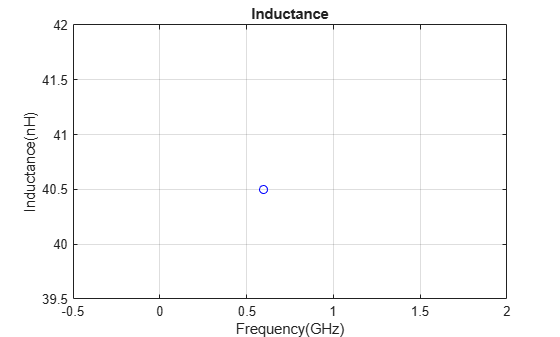inductance

Calculate inductance

Description

example

inductance(object,frequency) calculates and plots the inductance of an inductor over the specified frequency.

l = inductance(object,frequency) calculates the inductance of an inductor over the specified frequency.

Examples

collapse all

Create a hexagonal spiral inductor with default properties.

inductor = spiralInductor(SpiralShape='Hexagon');
show(inductor)Calculate the inductance of the inductor at 600 MHz.

inductance(inductor,600e6)l = inductance(inductor,600e6)
l = 3.6937e-08

Input Arguments

collapse all

Spiral inductor, specified as an spiralInductor object.

Data Types: char | string

Frequency to calculate inductance, specified as a nonnegative scalar or vector in hertz.

Data Types: double

Output Arguments

collapse all

Inductance of the inductor, returned as a scalar or vector in henries.

Data Types: double